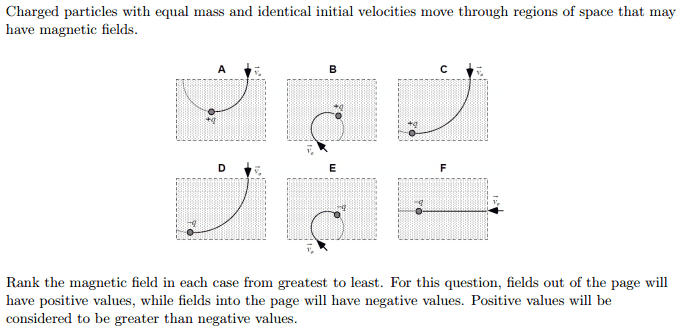Magnetic fields, charged particles and directions

Homework Statement

[/B]
Charged particles with equal mass and identical initial velocities move through regions of space that may have magnetic fields. Rank the magnetic field in each case from greatest to least. For this question, fields out of the page will have positive values, while fields into the page will have negative values. Positive values will be considered to be greater than negative values.Homework Equations

Right hand rule: Cross V(vector) into B(vector), the new vector VxB will point in the direction of your thumb.

The Attempt at a Solution

I am not sure I understand what the graphs are depicting. It wants me to find the direction of B but I only know how to find the direction of Fʙ which is VxB using the right hand rule. Can I somehow decompose that rule to find B given Fʙ?

My attempt, I assumed that if q is deflected to the left from the right then B comes out of the page:

A>C=D>F>B=E

I am not sure though maybe someone could help clear this up or tell me if I am correct that would helpful.

A charged particle moving in a direction of uniform magnetic field experiences a force.
At every point, this force is perpendicular to the direction of motion since vxB is perpendicular to v.
What motion will you get in such case where you have a constant force acting perpendicular to velocity of particle every time?

So then in A it points out of the page correct and B it point into the page. C out, D out E in and F out?

Last edited:
No. The magnetic field in A goes into the plane of paper (diagram is not clear). So just as the particle enters the region, it experience a force towards left side. So it turns a little.
Again the force changes direction since the direction of particle changes. But it always acts towards a central point
Such a motion is called circular motion.
For a moment forget the cases. When a charged particle executes circular motion in a uniform region of magnetic field, the magnetic force provides centripetal force.
Find the radius of circular path.

Last edited:
No. The magnetic field in A goes into the plane of paper (diagram is not clear). So just as the particle enters the region, it experience a force towards left side. So it turns a little.
Again the force changes direction since the direction of particle changes. But it always acts towards a central point
Such a motion is called circular motion.
For a moment forget the cases. When a charged particle executes circular motion in a uniform region of magnetic field, the magnetic force provides centripetal force.
Find the radius of circular path.

So then the stronger the magnetic force the further the particle will be pushed inward? Ie. B=E>A>D=C>F

correct!
since the force due to magnetic field provides the centripetal force,
$$\frac{mv^2}{R}=qvB$$
$$R=\frac{mv}{qB}$$
now we know as B increases, R decreases. So greater the magnetic field, lesser the radius of circular motion of particle.
For a negetive charges to move toward the left side, B as to be inwards (negative).
First assign signs to the magnetic field for each case.
B=E>A>D=C>F
this is wrong since you did not consider the sign.

correct!
since the force due to magnetic field provides the centripetal force,
$$\frac{mv^2}{R}=qvB$$
$$R=\frac{mv}{qB}$$
now we know as B increases, R decreases. So greater the magnetic field, lesser the radius of circular motion of particle.
For a negetive charges to move toward the left side, B as to be inwards (negative).
First assign signs to the magnetic field for each case.

this is wrong since you did not consider the sign.

I guess I am still a bit confused about why moving left makes B negative and moving right makes B positive. I am assuming it has something to do with the charge on the particle? Knowing that I have changed my answer too: B=E>A>D=C>F

I guess I am still a bit confused about why moving left makes B negative and moving right makes B positive. I am assuming it has something to do with the charge on the particle? Knowing that I have changed my answer too: B=E>A>D=C>F
Read the question properly.remember that Magnetic field is a vector. Yes. It depends on the charge. Due to negative charge, your -qvB and not qvB. Think again.

Don't you agree that -5 is less than zero? Try again. You are almost there. For the case of undeviated path, B is zero. So that cas will be I the middle of the order.

Don't you agree that -5 is less than zero? Try again. You are almost there. For the case of undeviated path, B is zero. So that cas will be I the middle of the order.

Okay so I did not notice the charge changed signs. So here I believe is the correct answer: B>A>C>F>E>D. Where in cases A-C the E field comes out of the page. The opposite being true for the rest of the cases.

Okay so I did not notice the charge changed signs. So here I believe is the correct answer: B>A>C>F>E>D. Where in cases A-C the E field comes out of the page. The opposite being true for the rest of the cases.
One more correction. For case D magnetic field has negetive sign but lesser magnitude. Magnetic field in E is also negetive but with higher magnitude due to lesser radius. And you know yhat -2 > -5. So, D>E